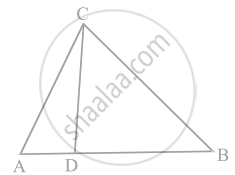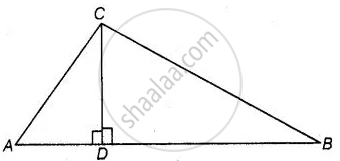# In the figure, if ∠ACB = ∠CDA, AC = 8 cm and AD = 3 cm, find BD. - Mathematics

Sum

In the figure, if ∠ACB = ∠CDA, AC = 8 cm and AD = 3 cm, find BD.#### Solution

Given, AC = 8 cm, AD = 3cm

And ∠ACB = ∠CDA

From figure, ∠CDA = 90°

∠ACB = ∠CDA = 90°⇒ (8)2 = (3)2 + (CD)2

⇒ 64 - 9 = CD^2

⇒ CD = sqrt(55) cm

∠DBC = ∠DCA    .....[Each equal to 90° – ∠A]

Then, (CD)/(BD) = (AD)/(CD)

⇒ CD^2 = AD xx BD

∴ BD = (CD^2)/(AD) = (sqrt(155))^2/3 = 55/3 cm

Concept: Similarity of Triangles
Is there an error in this question or solution?

#### APPEARS IN

NCERT Mathematics Exemplar Class 10
Chapter 6 Triangles
Exercise 6.3 | Q 13 | Page 69
Share